## Partnership

Partnership is a business in which two or more persons come together with a motive to divide the profits. For this purpose we classify it into two types.

Simple Partnership
If the time period of investment of all the partners is same. Even if the investment are different, then that partnership is a simple partnership. The profit in this case is divided in the ratio of the investments

Compound Partnership
If the time period of investment of any one or more partners is different even if the investment are same then that partnership is compound partnership. In this case the profit is divided in the ratio of the products of the investments and respective time period.
• Partners who share the investment also share the profits or loss if any.
• Usually all the partners take active part in running the business. Sometimes one partner may invest his money but not take an active part in running the business. Such a partner is called sleeping partner. He leaves the task of running the business to the other partner. The partner who runs the business is called the working partner.
 Simple Partnership Compound Partnership The profit or loss is divided in the ratio of their investments. The Profit or loss is divided in the ratio of their monthly equivalent investments. If two partners A and B have entered a simple partnership then: Investment of A : Investment of B = Profit of A : Profit of B If two partners A and B have entered a compound partnership then : Monthly equivalent of A : Monthly equivalent of B = Profit of A : Profit of B Monthly equivalent of A : Monthly equivalent of B = Loss of A : Loss of B Investment of A : Investment of B = Loss of A : Loss of B Monthly equivalent investment is the product of capital and the number of months for which it is invested.

Example 1.A with Rs. 4000, B with Rs. 7000 and C with Rs. 12000 started a business. After a year if the combined profit of B and C is Rs. 3000 more than that of A. Find the total profit.
Solution: Ratio of investments of A : B : C = 4 : 7 : 12
Hence, (B + C) - A = (12 + 7 - 4) = 15
If (B + C) = A is Rs. 3000 then, Total profit =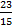x 3000 = Rs 4600
Example 2.A with Rs. 8000 and B with Rs. 11000 started a business. A gets 25% of the profit as salary being a working partner. Remaining is divided in the ratio of investments. If A gets Rs. 4300, find the Total Profit.
Solution: Let the total profit be x
Profit of A is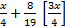= 4300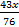= 4300 Therefore, x = Rs. 7600

Example 3. A with Rs. 4000 and B with Rs. 11000 started a business. 3 months later C joined with Rs. 7000, 2 months later A leaves. After 12 months if B gets Rs. 1320 as profit. Find the total profit of A & C
Solution: Ratio of Investment of A : B : C = 4 : 11 : 7
Ratio of time periods = 5 : 12 : 9
Ratio of Profits = 20 : 132 : 63
If B gets Rs. 132 then total profit of A & C = Rs. 83
If B gets Rs. 1320 then (A + C)’s profit = Rs. 830

Example 4. A with Rs. 8000 started a business. B joined later with Rs. 12000. After 18 months they divided the profit in ratio 2 : 1, when did B join?
Solution: Ratio of profits 2 : 1
Ratio of investment 2 : 3
Ratio of time periods =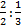= 1 := 3 : 1
B’s investment is forx 18 months = 6 months
B joined after 1 year.

Example 5.A invests Rs. 5000 and B invests Rs. 6000. A is a working partner. A receives 1/8 of the profit as salary and rest is divided in proportion of their capitals. If A gets Rs. 460 then find the total profit.
Solution: Ratio of investment is 5 : 6
Let the total profit be x
Share of A =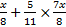= Rs. 460
=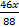= 460 Therefore, x = Rs. 880

Example 6.A, B, C enter into a partnership. A advances Rs. 600 for 8 months, B advances Rs. 2800 for 4 months, C advances Rs 2000 for 5 months. They earn a profit of Rs. 585 together. Share of C is
Solution: Ratio of investment (A : B : C) (600 : 2800 : 2000)
Ratio of time periods 8 : 4 : 5
Ratio of profits = 4800 : 11200 : 10000
= 12 : 28 : 25
Share of C =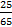x Rs. 585 = Rs. 225

Example 7.A invested Rs. 24000 for 8 months and investment of B is for 4 months. Annual ratio of profits is 3 : 4. Find the investment of B
Solution: Ratio of profit is 3 : 4
Ratio of time period is 2 : 1
Ratio of investment is:= 3 : 8
If investment of A = Rs. 24000
Therefore investment of B = Rs. 64000

Example 8. A and B rent a pasture for 20 months. A puts in 160 oxen for 14 months. How many can B put in for the remaining 6 months, if he pays half as much as again.
Solution: Rent ratio of A & B = 2 : 3 (half as much again means half more)
Time ratio = 14 : 6
Oxen ratio =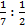= 2 : 7 160 : 560
Example 9. Investment ratio of A, B, C is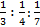for the same time period. If A gets Rs.560 as profit then how much does B get more than C.
Solution: Investment ratio is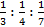= 28 : 21 : 12
If A gets Rs. 28 then (B - C) = Rs.9
If A gets Rs: 560 then (B - C) is 560 x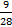= Rs. 180

Example 10.A, B & C enter into a partnership for 1 year A invests his money at the start. B invests twice the amount of A but after 6 months, C invests 3/2 times that of B after 8 months. If total profit = Rs. 12000. What is the Share of B ?
Solution: Investment ratio A : B : C = 1 : 2 : 3
Time period of investment = 12 : 6 : 4
Profit ratio is 1 : 1 : 1
Share of B =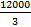= Rs. 4000Want to Know More
Please fill in the details below:

Name

ltr
item
Clear CTET - Coaching Institute for CTET, DSSSB and KVS: dsssb-quants-partnership-theory-part1
dsssb-quants-partnership-theory-part1